## 2.15  Projects

Exercise 2.20.  Free rigid body
Write and demonstrate a program that reproduces diagrams like figure 2.3 (section 2.9.2). Can you find trajectories that are asymptotic to the unstable relative equilibrium on the intermediate principal axis?

Exercise 2.21.  Rotation of Mercury
In the '60s it was discovered that Mercury has a rotation period that is precisely 2/3 times its orbital period. We can see this resonant behavior in the spin-orbit model problem, and we can also play with nudging Mercury a bit to see how far off the rotation rate can be and still be trapped in this spin-orbit resonance. If the mismatch in angular velocity is too great, Mercury's rotation is no longer resonantly locked to its orbit. Set= 0.026 and e = 0.2.

a.  Write a program for the spin-orbit problem so this resonance dynamics can be investigated numerically. You will need to know (or, better, show!) that f satisfies the equation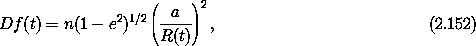with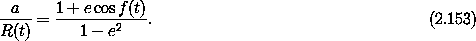Notice that n disappears from the equations if they are written in terms of a new independent variable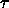= n t. Also notice that a and R(t) appear only in the combination a/R(t).

b.  Show that the 3:2 resonance is stable by numerically integrating the system when the rotation is not exactly in resonance and observing that the angle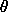- (3/2) nt oscillates.

c.  Find the range of initial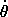for which this resonance angle oscillates.